Download Presentation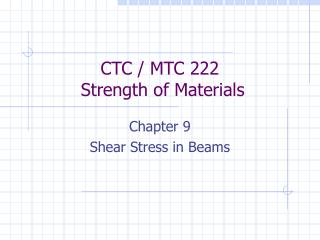CTC / MTC 222 Strength of Materials

# CTC / MTC 222 Strength of Materials - PowerPoint PPT Presentation

CTC / MTC 222 Strength of Materials. Chapter 9 Shear Stress in Beams. Chapter Objectives. List the situations where shear stress in a beam is likely to be critical. Compute the shear stress in a beam using the general shear formula.I am the owner, or an agent authorized to act on behalf of the owner, of the copyrighted work described.
Download Presentation## CTC / MTC 222 Strength of Materials

An Image/Link below is provided (as is) to download presentation

Download Policy: Content on the Website is provided to you AS IS for your information and personal use and may not be sold / licensed / shared on other websites without getting consent from its author.While downloading, if for some reason you are not able to download a presentation, the publisher may have deleted the file from their server.

- - - - - - - - - - - - - - - - - - - - - - - - - - E N D - - - - - - - - - - - - - - - - - - - - - - - - - -
Presentation Transcript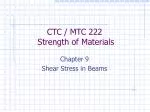### CTC / MTC 222 Strength of Materials

Chapter 9

Shear Stress in Beams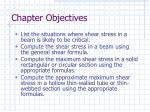Chapter Objectives
• List the situations where shear stress in a beam is likely to be critical.
• Compute the shear stress in a beam using the general shear formula.
• Compute the maximum shear stress in a solid rectangular or circular section using the appropriate formulas.
• Compute the approximate maximum shear stress in a hollow thin-walled tube or thin-webbed section using the appropriate formulas.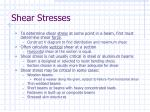Shear Stresses
• To determine shear stress at some point in a beam, first must determine shear force.
• Construct V diagram to find distribution and maximum shear.
• Often calculate vertical shear at a section
• Horizontal shear at the section is equal.
• Shear stress is not usually critical in steel or aluminum beams
• Beam is designed or selected to resist bending stress.
• Section chosen is usually more than adequate for shear
• Shear stress may be critical in some cases:
• Wooden beams
• Wood is weaker along the grain, subject to failure from horizontal shear
• Thin-webbed beams
• Short beams or beams with heavy concentrated loads
• Fasteners in built-up or composite beams
• Stressed skin structures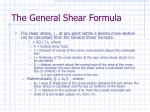The General Shear Formula
• The shear stress,  , at any point within a beams cross-section can be calculated from the General Shear Formula:
•  = VQ / I t, where
• V = Vertical shear force
• I = Moment of inertia of the entire cross-section about the centroidal axis
• t = thickness of the cross-section at the axis where shear stress is to be calculated
• Q = Statical moment about the neutral axis of the area of the cross-section between the axis where the shear stress is calculated and the top (or bottom) of the beam
• Q is also called the first moment of the area
• Mathematically, Q = AP ̅y̅ , where:
• AP = area of theat part of the cross-section between the axis where the shear stress is calculated and the top (or bottom) of the beam
• ̅y̅ = distance to the centroid of AP from the overall centroidal axis
• Units of Q are length cubed; in3, mm3, m3,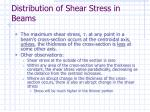Distribution of Shear Stress in Beams
• The maximum shear stress, , at any point in a beam’s cross-section occurs at the centroidal axis, unless, the thickness of the cross-section is less at some other axis.
• Other observations:
• Shear stress at the outside of the section is zero
• Within any area of the cross-section where the thickness is constant, the shear stress varies parabolically, decreasing as the distance from the centroid increases.
• Where an abrupt change in the thickness of the cross-section occurs, there is also an abrupt change in the shear stress
• Stress will be much higher in the thinner portion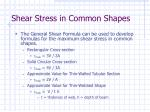Shear Stress in Common Shapes
• The General Shear Formula can be used to develop formulas for the maximum shear stress in common shapes.
• Rectangular Cross-section
• max = 3V / 2A
• Solid Circular Cross-section
• max = 4V / 3A
• Approximate Value for Thin-Walled Tubular Section
• max ≈ 2V / A
• Approximate Value for Thin-Webbed Shape
• max ≈ V / t h
• t = thickness of web, h = depth of beam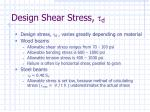Design Shear Stress, d
• Design stress, d , varies greatly depending on material
• Wood beams
• Allowable shear stress ranges from 70 - 100 psi
• Allowable bending stress is 600 – 1800 psi
• Allowable tension stress is 400 – 1000 psi
• Failure is often by horizontal shear, parallel to grain
• Steel beams
• d = 0.40 SY
• Allowable stress is set low, because method of calculating stress (max ≈ V / t h ) underestimates the actual stress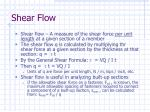Shear Flow
• Shear flow – A measure of the shear force per unit length at a given section of a member
• The shear flow q is calculated by multiplying thr shear force at a given section by the thickness at that section: q =  t
• By the General Shear Formula: = VQ / I t
• Then q =  t = VQ / I
• Units of q are force per unit length, N / m, kips / inch, etc.
• Shear flow is useful in analyzing built-up sections
• If the allowable shear force on a fastener, Fsd , is known, the maximum allowable spacing of fasteners required to connect a component of a built-up section, smax , can be calculated from: smax= Fsd / q